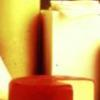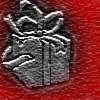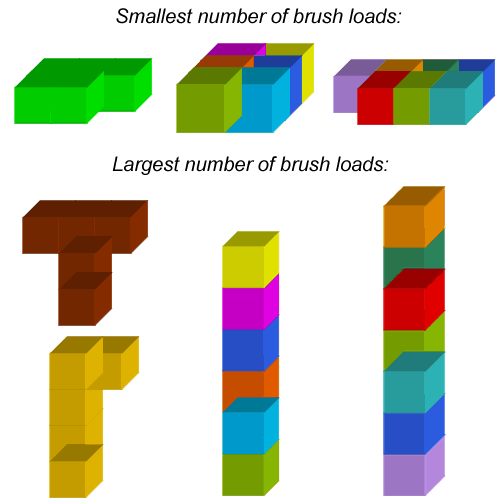#### You may also like### Cubes

How many faces can you see when you arrange these three cubes in different ways?### The Big Cheese

Investigate the area of 'slices' cut off this cube of cheese. What would happen if you had different-sized block of cheese to start with?### Wrapping Presents

Choose a box and work out the smallest rectangle of paper needed to wrap it so that it is completely covered.

##### Age 7 to 11Challenge Level

Well done to everybody who had a go at this activity.

Lots of children said that the smallest number of brush loads needed was 15 and the largest number was 21. Dana from Twyford School in the UK made arrangements of cubes that used all of the numbers of brush loads between 15 and 21, and she even found a way to make an arrangement using 22 brush loads! Have a look at Dana's solution to see how she did it.

Callum and Josh submitted some ways of making the largest number of brush loads and the smallest number of brush loads for 5, 6 and 7 cubes:Lan explored the pattern for greater numbers of cubes, as did Rohaan from New Zealand. Lan describes the method used:

From counting cubes, we find:
With 5 cubes, the least number of BLs is 15, and the largest number of BLs is 21.
With 6 cubes, the least number of BLs is 16, and the largest number of BLs is 25.
With 7 cubes, the least number of BLs is 19, and the largest number of BLs is 29.
With 8 cubes, the least number of BLs is 20, and the largest number of BLs is 33.
With 9 cubes, the least number of BLs is 23, and the largest number of BLs is 37.

We remark the pattern:
With 5 cubes, the least number is 15, and the largest number is 21.
With 6 cubes, the least number is 15+1 = 16, and the largest number is 21+4 = 25.
With 7 cubes, the least number is 16+3 = 19, and the largest number is 25+4 = 29.
With 8 cubes, the least number is 19+1 = 20, and the largest number is 29+4 = 33.
With 9 cubes, the least number is 20+3 = 23, and the largest number is 33+4 = 37.

This is interesting, isn't it? If we think about the least number of BLs, we are trying to make as close to a rectangular arrangement of cubes as possible. This helps us to see why we only add one face going from an odd to an even number of cubes, but three faces going from an even to an odd.

However, is a rectangular array always the best for minimising the number of brush loads? If you had 9 cubes, how about making a square arrangement? How many brush loads of paint would you need then?

Ci Hui Minh Ngoc from Kong Hwa School in Singapore worked out the number of brush loads needed for every number of cubes from 1 to 7, thinking particularly about the ways of arranging the cubes to give the smallest and largest numbers of brush loads. Have a look at Ci Hui Minh Ngoc's solution to see if you agree with their ideas. The formula under each diagram represents the number of 'covered' faces, i.e. the number of faces that don't need painting, compared to the total number of faces on each of the cubes.

Ci Hui Minh Ngoc says that when the cubes are arranged in a tall tower, the number of 'covered' faces in the arrangement with n cubes can be written as (n - 1) x 2 + 1, compared to the total number of faces on all of the cubes which would be 6n.

Rohaan's solution says that the largest number of brush loads is always (4 x number of cubes) + 1. Does this tie in with what Ci Hui Minh Ngoc says about the number of covered faces?

Rohaan also worked out that the smallest number of brush loads depends on whether the number of cubes is odd or even:

Odd: (n - 5) x 2 + 15
Even: (n - 6) x 2 + 16

Does this work for a square arrangement too?